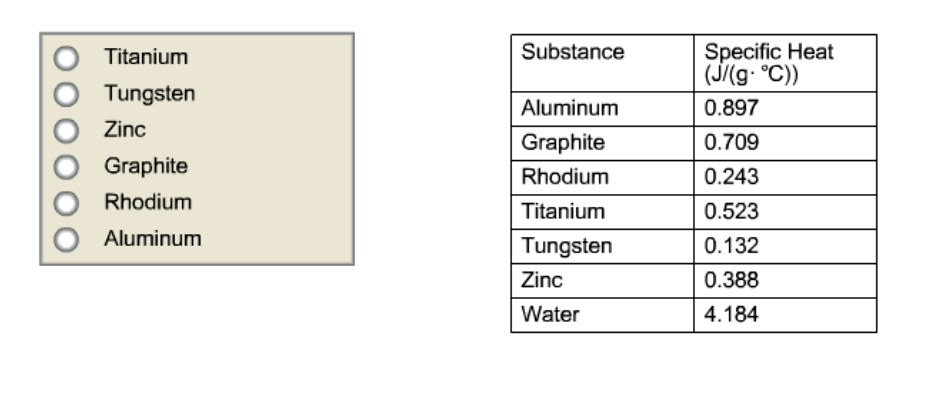# Problem: A hot lump of 106.2 g of an unknown substance initially at 153.2°C is placed in 35.0 mL of water initially at 25.0°C and allowed to reach thermal equilibrium. The final temperature of the system is 53.2°C. What is the identity of the unknown substance? Assume no heat is lost to the surroundings.

###### FREE Expert Solution
82% (453 ratings)
###### FREE Expert Solution

We’re being asked to determine the identity of the unknown substance in the given system.

Recall that heat (q) can be calculated using the following equation:

$\overline{){\mathbf{q}}{\mathbf{=}}{\mathbf{mc}}{\mathbf{∆}}{\mathbf{T}}}$

q = heat, J

+qabsorbs heat
–qloses heat

m = mass (g)
c = specific heat capacity = J/(g·°C)
ΔT = Tf – Ti = (°C)

Based on the given system:

82% (453 ratings)###### Problem Details

A hot lump of 106.2 g of an unknown substance initially at 153.2°C is placed in 35.0 mL of water initially at 25.0°C and allowed to reach thermal equilibrium. The final temperature of the system is 53.2°C. What is the identity of the unknown substance? Assume no heat is lost to the surroundings.What scientific concept do you need to know in order to solve this problem?

Our tutors have indicated that to solve this problem you will need to apply the Calorimetry concept. You can view video lessons to learn Calorimetry. Or if you need more Calorimetry practice, you can also practice Calorimetry practice problems.

What is the difficulty of this problem?

Our tutors rated the difficulty ofA hot lump of 106.2 g of an unknown substance initially at 1...as medium difficulty.

How long does this problem take to solve?

Our expert Chemistry tutor, Dasha took 8 minutes and 17 seconds to solve this problem. You can follow their steps in the video explanation above.

What professor is this problem relevant for?

Based on our data, we think this problem is relevant for Professor Giles' class at VCU.